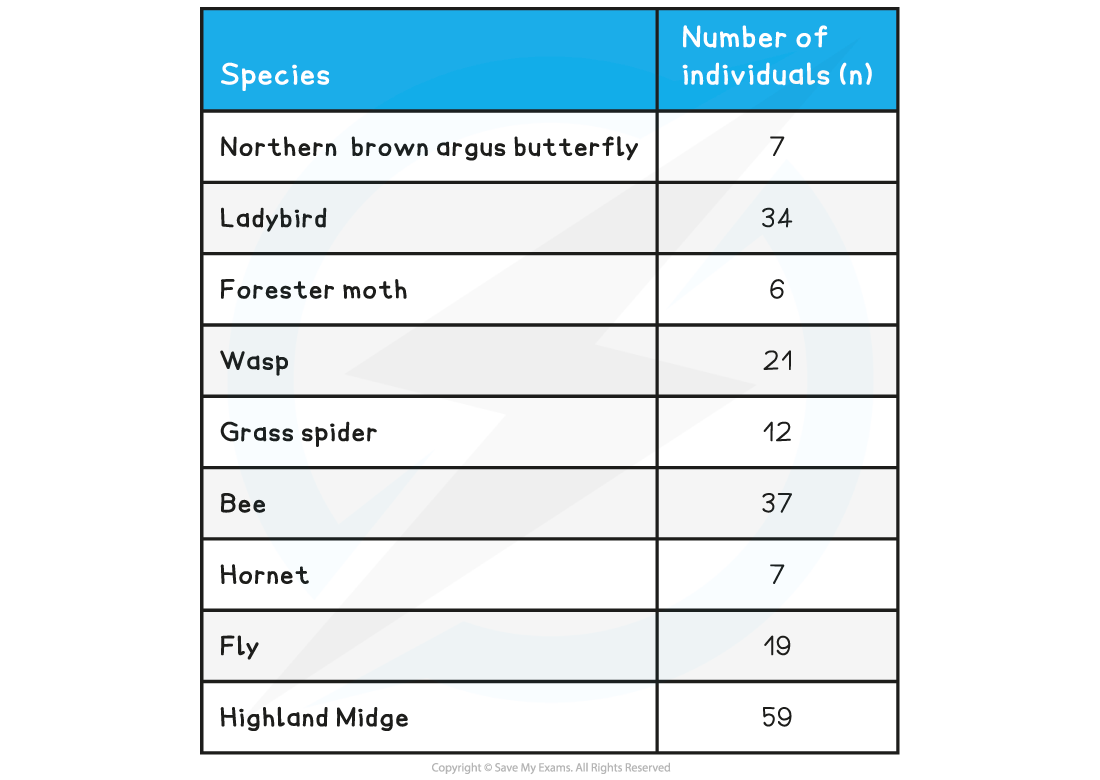# AQA A Level Biology复习笔记4.6.3 Index of Diversity

### The Index of Diversity

• Once the abundance of different species in an area has been recorded the results can be used to calculate the species diversity or biodiversity for that area
• Species diversity looks at the number of different species in an area but also the evenness of abundance across the different species
• An index of diversity is a measurement that describes the relationship between the number of species present and how each species contributes to the total number of organisms that are present in that community
• For example, habitat A has 10 different plant species and habitat B has 7 different plant species
• Habitat A would have a greater species richness score than habitat B
• However, in habitat A there is only one individual of each plant species present (10 individuals present) while in habitat B there are over 20 individuals of each species present (over 140 individuals present)
• Habitat B would have a greater index of diversity than habitat A

#### The index of diversity formula

• The formula is:• Where:
• n = total no. of organisms for a single species in the community
• N = total no. of organisms in the community
• Σ = sum of

•  The larger the number obtained, the higher the level of diversity

### Maths Skill: Calculating the Index of Diversity• To calculate:
• Step 1: Calculate N(N-1) to find value A
• Step 2: Calculate n(n-1) for each species
• Step 3: Add these numbers together to find value B
• Step 4: Divide value A by value B

• The possible values of index diversity are significant:
• The larger the number obtained, the higher the levels of diversity

#### Worked Example

Samples of different insect species in a back garden were collected using sweep nets and identification keys. Use the data recorded to calculate the index of diversity.The formula is:Step one:       Calculate N(N-1) to find value A

N(N-1) = 202(202-1) = 202(201) = 40602

Step two:       Calculate n(n-1) for each species

Step three:     Add these numbers together to find value BStep four:      Divide value A by value B

40602÷ 6884 = 5.898

Index of Diversity,d = 5.90

#### Exam Tip

Remember, you will be provided with the formula in the exam.